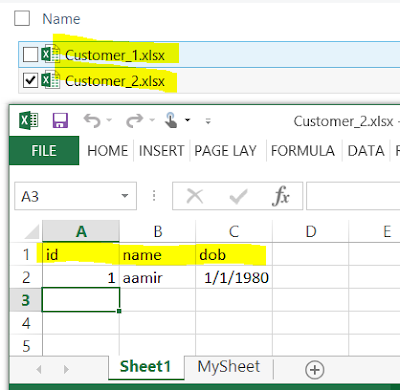### C# - Import Multiple Excel Files with Multiple Sheets to Single SQL Server Table in C#

You are working as C# or dot net developer. You need to write a program that should be able to load single or multiple excel files with single or multiple excel sheets to single SQL Server table from a folder. All the sheets have the same columns.

The Excel file names and excel sheet names are not constant. They can change any time.

Here are couple of sample files those I created for test the below program.How to import data from multiple excel files with single or multiple excel sheets to SQL Server Table in C#

Let's create SQL Server Table first before we use it in our C# program.

```CREATE TABLE [dbo].[tblCustomer](
[id] int NULL,
[name] varchar(100) NULL,
[dob] date NULL
) ```

I used below C# code to create Console Application.

```using System;
using System.Collections.Generic;
using System.Linq;
using System.Text;
using System.IO;
using System.Data;
using System.Data.OleDb;
using System.Data.SqlClient;

namespace TechBrothersIT.com_CSharp_Tutorial
{
class Program
{
static void Main(string[] args)
{
//the datetime and Log folder will be used for error log file in case error occured
string datetime = DateTime.Now.ToString("yyyyMMddHHmmss");
string LogFolder = @"C:\Log\";
try
{
//Provide the Source Folder path where excel files are present
String FolderPath = @"C:\Source\";
//Provide the Database Name
string DatabaseName = "TechbrothersIT";
//Provide the SQL Server Name
string SQLServerName = "(local)";
//Provide the table name in which you want to load excel sheet's data
String TableName = @"tblCustomer";
//Provide the schema of table
String SchemaName = @"dbo";

//Create Connection to SQL Server Database
SqlConnection SQLConnection = new SqlConnection();
SQLConnection.ConnectionString = "Data Source = "
+ SQLServerName + "; Initial Catalog ="
+ DatabaseName + "; "
+ "Integrated Security=true;";

var directory = new DirectoryInfo(FolderPath);
FileInfo[] files = directory.GetFiles();

//Declare and initilize variables
string fileFullPath = "";

//Get one Book(Excel file at a time)
foreach (FileInfo file in files)
{
fileFullPath = FolderPath + "\\" + file.Name;

//Create Excel Connection
string ConStr;
string HDR;
HDR = "YES";
ConStr = "Provider=Microsoft.ACE.OLEDB.12.0;Data Source="
+ fileFullPath + ";Extended Properties=\"Excel 12.0;HDR=" + HDR + ";IMEX=0\"";
OleDbConnection cnn = new OleDbConnection(ConStr);

//Get Sheet Name
cnn.Open();
DataTable dtSheet = cnn.GetOleDbSchemaTable(OleDbSchemaGuid.Tables, null);
string sheetname;
sheetname = "";

//Loop through each sheet
foreach (DataRow drSheet in dtSheet.Rows)
{
if (drSheet["TABLE_NAME"].ToString().Contains("\$"))
{
sheetname = drSheet["TABLE_NAME"].ToString();

//Get data from Excel Sheet to DataTable
OleDbConnection Conn = new OleDbConnection(ConStr);
Conn.Open();
OleDbCommand oconn = new OleDbCommand("select * from [" + sheetname + "]", Conn);
DataTable dt = new DataTable();
Conn.Close();

SQLConnection.Open();
//Load Data from DataTable to SQL Server Table.
using (SqlBulkCopy BC = new SqlBulkCopy(SQLConnection))
{
BC.DestinationTableName = SchemaName + "." + TableName;
foreach (var column in dt.Columns)
BC.WriteToServer(dt);
}
SQLConnection.Close();

}
}
}
}
catch (Exception exception)
{
// Create Log File for Errors
using (StreamWriter sw = File.CreateText(LogFolder
+ "\\" + "ErrorLog_" + datetime + ".log"))
{
sw.WriteLine(exception.ToString());

}

}

}
}
}```

I executed above C# Console Application and it loaded data from multiple excel files with multiple sheets to SQL Server Table.How to import multiple excel files with multiple Sheets to SQL Server Table in C#

1.2.